# Carbonic acid

Updated on
Covid-19
 Formula  H2CO3Molar mass  62.03 g/molSoluble in  Water IUPAC ID  Carbonic acidDensity  1.67 g/cm³Related compounds  Acetone Urea Carbonyl fluoride

## H2co3 lewis structure how to draw the lewis structure for carbonic acid

Carbonic acid is a chemical compound with the chemical formula H2CO3 (equivalently OC(OH)2). It is also a name sometimes given to solutions of carbon dioxide in water (carbonated water), because such solutions contain small amounts of H2CO3. In physiology, carbonic acid is described as volatile acid or respiratory acid, because it is the only acid excreted as a gas by the lungs. It plays an important role in the bicarbonate buffer system to maintain acid–base homeostasis.

## Contents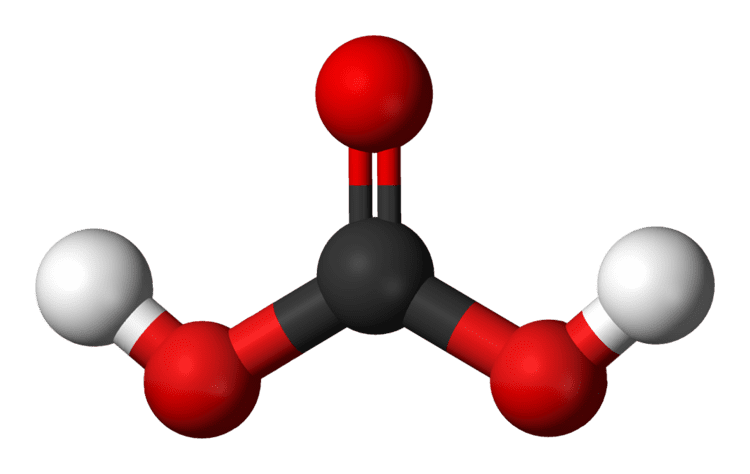Carbonic acid, which is a weak acid, forms two kinds of salts, the carbonates and the bicarbonates. In geology, carbonic acid causes limestone to dissolve producing calcium bicarbonate which leads to many limestone features such as stalactites and stalagmites.It was long believed that carbonic acid could not exist as a pure compound. However, in 1991 it was reported that NASA scientists had succeeded in making solid H2CO3 samples.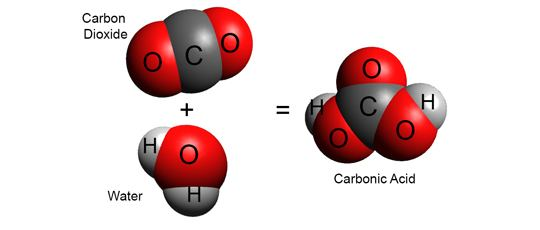## Chemical equilibrium

When carbon dioxide dissolves in water it exists in chemical equilibrium producing carbonic acid:

CO2 + H2O ⇌ H2CO3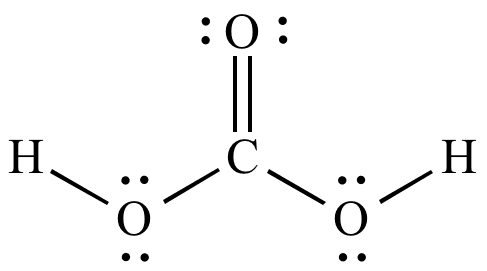The hydration equilibrium constant at 25 °C is called Kh, which in the case of carbonic acid is [H2CO3]/[CO2] ≈ 1.7×10−3 in pure water and ≈ 1.2×10−3 in seawater. Hence, the majority of the carbon dioxide is not converted into carbonic acid, remaining as CO2 molecules. In the absence of a catalyst, the equilibrium is reached quite slowly. The rate constants are 0.039 s−1 for the forward reaction (CO2 + H2O → H2CO3) and 23 s−1 for the reverse reaction (H2CO3 → CO2 + H2O). The addition of two molecules of water to CO2 would give orthocarbonic acid, C(OH)4, which exists only in minute amounts in aqueous solution.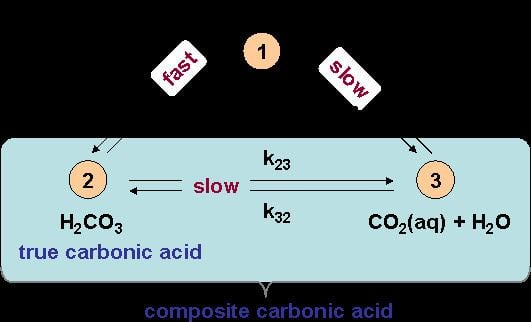Addition of base to an excess of carbonic acid gives bicarbonate (hydrogen carbonate). With excess base, carbonic acid reacts to give carbonate salts.

## Role of carbonic acid in blood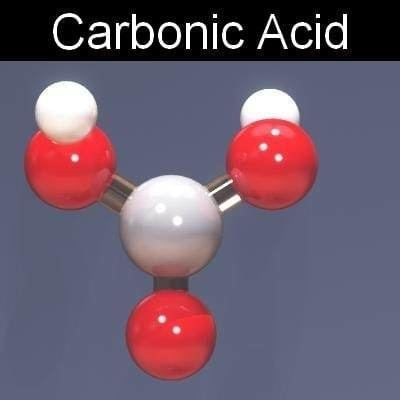Bicarbonate is an intermediate in the transport of CO2 out of the body via respiratory gas exchange. The hydration reaction of CO2 is generally very slow in the absence of a catalyst, but red blood cells contain carbonic anhydrase, which increases the reaction rate, producing bicarbonate (HCO3) dissolved in the blood plasma. This catalysed reaction is reversed in the lungs, where it converts the bicarbonate back into CO2 and allows it to be expelled. This equilibration plays an important role as a buffer in mammalian blood. A 2016 theoretical report suggests that carbonic acid may play a pivotal role in protonating various nitrogen bases in blood serum.

## Role of carbonic acid in ocean chemistry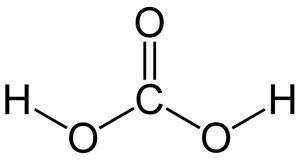The oceans of the world have absorbed almost half of the CO2 emitted by humans from the burning of fossil fuels.  It has been theorized that the extra dissolved carbon dioxide has caused the ocean's average surface pH to shift by about −0.1 unit from pre-industrial levels. This theory is known as ocean acidification, even though the ocean remains basic.

## Acidity of carbonic acidCarbonic acid is a polyprotic acid — specifically it is diprotic meaning it has two protons which may dissociate from the parent molecule. Thus, there are two dissociation constants, the first one for the dissociation into the bicarbonate (also called hydrogen carbonate) ion HCO3:

H2CO3 ⇌ HCO3 + H+ Ka1 = 2.5×10−4; pKa1 = 3.6 at 25 °C.

Care must be taken when quoting and using the first dissociation constant of carbonic acid. In aqueous solution, carbonic acid exists in equilibrium with carbon dioxide, and the concentration of H2CO3 is much lower than the concentration of CO2. In many analyses, H2CO3 includes dissolved CO2 (referred to as CO2(aq)), H2CO3* is used to represent the two species when writing the aqueous chemical equilibrium equation. The equation may be rewritten as follows:

H2CO3* ⇌ HCO3 + H+ Ka(app) = 4.47×10−7; pK(app) = 6.35 at 25 °C and ionic strength = 0.0

Whereas this apparent pKa is quoted as the dissociation constant of carbonic acid, it is ambiguous: it might better be referred to as the acidity constant of dissolved carbon dioxide, as it is particularly useful for calculating the pH of CO2-containing solutions. A similar situation applies to sulfurous acid (H2SO3), which exists in equilibrium with substantial amounts of unhydrated sulfur dioxide.

The second constant is for the dissociation of the bicarbonate ion into the carbonate ion CO32−:

HCO3 ⇌ CO32− + H+ Ka2 = 4.69×10−11; pKa2 = 10.329 at 25 °C and ionic strength = 0.0

The three acidity constants are defined as follows: K a 1 = [ H + ] [ HCO 3 ] [ H 2 CO 3 ] K a (app) = [ H + ] [ HCO 3 ] [ H 2 CO 3 ] + [ CO 2 (aq) ] K a 2 = [ H + ] [ CO 3 2 ] [ HCO 3 ]

## pH and composition of carbonic acid solutions

At a given temperature, the composition of a pure carbonic acid solution (or of a pure CO2 solution) is completely determined by the partial pressure p CO 2 of carbon dioxide above the solution. To calculate this composition, account must be taken of the above equilibria between the three different carbonate forms (H2CO3, HCO3 and CO32−) as well as of the hydration equilibrium between dissolved CO2 and H2CO3 with constant K h = [ H 2 CO 3 ] [ CO 2 ] (see above) and of the following equilibrium between the dissolved CO2 and the gaseous CO2 above the solution:

CO2(gas) ⇌ CO2(dissolved) with [ CO 2 ] p CO 2 = 1 k H , where kH = 29.76 atm/(mol/L) (Henry constant) at 25 °C.

The corresponding equilibrium equations together with the [ H + ] [ OH ] = 10 14 relation and the charge neutrality condition [ H + ] = [ OH ] + [ HCO 3 ] + 2 [ CO 3 2 ] result in six equations for the six unknowns [CO2], [H2CO3], [H+], [OH], [HCO3] and [CO32−], showing that the composition of the solution is fully determined by p CO 2 . The equation obtained for [H+] is a cubic whose numerical solution yields the following values for the pH and the different species concentrations:

• We see that in the total range of pressure, the pH is always largely lower than pKa2 so that the CO32− concentration is always negligible with respect to HCO3 concentration. In fact CO32− plays no quantitative role in the present calculation (see remark below).
• For vanishing p CO 2 , the pH is close to the one of pure water (pH = 7) and the dissolved carbon is essentially in the HCO3 form.
• For normal atmospheric conditions ( p CO 2 = 3.5 × 10 4 atm), we get a slightly acid solution (pH = 5.7) and the dissolved carbon is now essentially in the CO2 form. From this pressure on, [OH] becomes also negligible so that the ionized part of the solution is now an equimolar mixture of H+ and HCO3.
• For a CO2 pressure typical of the one in carbonated drink bottles ( p CO 2 ~ 2.5 atm), we get a relatively acid medium (pH = 3.7) with a high concentration of dissolved CO2. These features contribute to the sour and sparkling taste of these drinks.
• Between 2.5 and 10 atm, the pH crosses the pKa1 value (3.60), giving a dominant H2CO3 concentration (with respect to HCO3) at high pressures.
• A plot of the equilibrium concentrations of these different forms of dissolved inorganic carbon (and which species is dominant), as a function of the pH of the solution, is known as a Bjerrum plot.
• Remark

As noted above, [CO32−] may be neglected for this specific problem, resulting in the following very precise analytical expression for [H+]:

[ H + ] ( 10 14 + K h K a 1 k H p CO 2 ) 1 / 2 .

## Pure carbonic acid

It was long believed that carbonic acid could not exist as a pure compound. However, in 1991 scientists at NASA's Goddard Space Flight Center (USA) succeeded in making solid H2CO3 samples. They did so by exposing a frozen mixture of water and carbon dioxide to high-energy proton radiation, and then warming to remove the excess water. The carbonic acid that remained was characterized by infrared spectroscopy. The fact that the carbonic acid was prepared by irradiating a solid H2O + CO2 mixture, or even by irradiation of dry ice alone, has given rise to suggestions that H2CO3 might be found in outer space or on Mars, where frozen ices of H2O and CO2 are found, as well as cosmic rays. It was announced in 1993 that solid carbonic acid had been created by a cryogenic reaction of potassium bicarbonate and HCl dissolved in methanol. Later work showed that in fact the methyl ester had been formed, but other methods were successful. Theoretical calculations showed that a single molecule of water can catalyze the decomposition of a gas-phase carbonic acid molecule to carbon dioxide and water. In the absence of water, the dissociation of gaseous carbonic acid has been predicted to be very slow, with a half-life of 180,000 years. This only applies if the molecules are few and far between, because it has also been predicted that gas-phase carbonic acid will catalyze its own decomposition by forming dimers which then break apart into two molecules each of water and carbon dioxide.

For a while it was thought that there were two polymorphs of solid carbonic acid, called α and β. The polymorph denoted beta-carbonic acid was prepared by heating alternating layers of glassy aqueous solutions of bicarbonate and acid in vacuum, which causes protonation of the bicarbonate, followed by removal of the solvent. The previously suggested alpha-carbonic acid, which was prepared by the same technique using methanol rather than water as a solvent was later shown to be a monomethyl ester CH3OCOOH.

## References

Similar Topics
The Sisters (1938 film)
True Noon (film)
Mason Morfit
Topics

 B i Link H2
 L